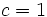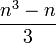# Free nilpotent group

## Definition

Suppose$c$ is a positive integer. The free nilpotent group of class$c$ on a set$S$ can be defined in the following equivalent ways:

1. It is the group$F/\gamma_{c+1}(F)$ where$F$ is the free group on$S$ and$\gamma_{c+1}(F)$ is the$(c+1)^{th}$ member of the lower central series of$F$.
2. It is the reduced free group corresponding to the subvariety of groups of nilpotency class$c$ in the variety of groups.

## Arithmetic functions

The quotient groups$\gamma_r(F)/\gamma_{r+1}(F)$ are free abelian groups of rank given by the formula for dimension of graded component of free Lie algebra. Explicitly, if$S$ has size$n$, this is:$\frac{1}{r} \sum_{d|r} \mu(d)n^{r/d}$

Function Value Explanation
nilpotency class$c$ by construction (note that the class being exactly$c$ is relatively easy to check, and follows also from the existence of any group with class exactly$c$).
Hirsch length$\sum_{r=1}^c \left(\frac{1}{r} \sum_{d|r} \mu(d)n^{r/d}\right)$ We have to add up the ranks of all the successive factor groups in the lower central series.
minimum size of generating set$n$ by construction

## Group properties

Property Meaning Satisfied? Explanation
nilpotent group Yes
UL-equivalent group upper central series and lower central series coincide Yes

## Particular cases

• If$c = 1$, we get a free abelian group. If$S$ is finite and has size$n$, this is the group$\mathbb{Z}^n$.
• If$c = 2$ and$S$ has size two, we get unitriangular matrix group:UT(3,Z).

### Hirsch lengths

We work with the group with$n$ generators.

Nilpotency class$c$ Rank of center as a free abelian group (given by formula for dimension of graded component of free Lie algebra) Hirsch length (equals sum of rank of center and Hirsch length of preceding group)
1$n$$n$
2$\frac{n^2 - n}{2}$$\frac{n(n + 1)}{2}$
3$\frac{n^3 - n}{3}$$\frac{n(n+1)(2n+1)}{6}$
4$\frac{n^4 - n^2}{4}$$\frac{n(n+1)(3n^2 + n + 2)}{12}$